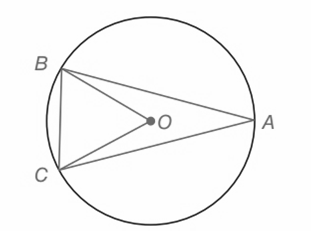Chapter 6.CT, Problem 2CT### Elementary Geometry for College St...

6th Edition
Daniel C. Alexander + 1 other
ISBN: 9781285195698

#### Solutions

Chapter
Section### Elementary Geometry for College St...

6th Edition
Daniel C. Alexander + 1 other
ISBN: 9781285195698
Textbook Problem
27 views

# a) If m B C ⌢ = 69 ° , then m ∠ B O C = _____________.b) If m B C ⌢ = 64 ° , then m ∠ B A C = _______________.To determine

(a)

To find:

The measure of mBOC

Explanation

Given:

mBC=69°

Approach:

1) In a circle the measure of a central angle is equal to the measure of the arc that it intercepts.

2) In a circle the measure of an interior angle is equal to one-half of the arc that it intercepts.

Calculation:

Consider the given circle,

mBC=69°.

BC is the arc that intercept the central angle

To determine

(b)

To find:

The measure of mBAC

### Still sussing out bartleby?

Check out a sample textbook solution.

See a sample solution

#### The Solution to Your Study Problems

Bartleby provides explanations to thousands of textbook problems written by our experts, many with advanced degrees!

Get Started

#### 11. Maximize subject to

Mathematical Applications for the Management, Life, and Social Sciences

#### True or False: converges.

Study Guide for Stewart's Multivariable Calculus, 8th

#### Identify the basic features of a between-subjects research design.

Research Methods for the Behavioral Sciences (MindTap Course List)